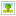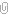# JS正则匹配表达式语法汇总（附实例）

. 查找单个字符，除了换行和行结束符
\w 查找单词字符
\W 查找非单词字符
\d 查找数字
\D 查找非数字字符
\s 查找空白字符
\S 查找非空白字符
\b 匹配单词边界
\B 匹配非单词边界
\0 查找 NUL字符
\n 查找换行符
\f 查找换页符
\r 查找回车符
\t 查找制表符
\v 查找垂直制表符
\xxx 查找以八进制数 xxxx 规定的字符
\xdd 查找以十六进制数 dd 规定的字符
\uxxxx 查找以十六进制 xxxx规定的 Unicode 字符

```
var r = /\x61/;
var s = "JavaScript";
var a = s.match(s);

```

```
var r = /\141/;
var s = "JavaScript";
var a = s.match(r);

```

```
var r = "/\u0061/";
var s = "JavaScript";
var a = s.match(s);

```

```
var r = new RegExp("\\u0061");

```

RegExp() 构造函数的参数只接受字符串，而不是字符模式。在字符串中，任意字符加反斜杠还表示字符本身，如字符串“\u”就被解释为 u 本身，所以对于“\u0061”字符串来说，在转换为字符模式时，就被解释为“u0061”，而不是“\u0061”，此时反斜杠就失去转义功能。解决方法：在字符 u 前面加双反斜杠。

[abc]：查找方括号内任意一个字符。
[^abc]：查找不在方括号内的字符。
[0-9]：查找从 0 至 9 范围内的数字，即查找数字。
[a-z]：查找从小写 a 到小写 z 范围内的字符，即查找小写字母。
[A-Z]：查找从大写 A 到大写 Z 范围内的字符，即查找大写字母。
[A-z]：查找从大写 A 到小写 z 范围内的字符，即所有大小写的字母。

```
var r = /[\u0000-\u00ff]/g;

```

```
var r = /[^\u0000-\u00ff]/g;

```

```
var r = /[a-zA-Z0-9]/g;

```

```
var r = /[\u0030-\u0039]/g;

```

```
var r = /[\u0041-\u004A]/g;

```

```
var r = /[\u0061-\u007A]/g;

```

```
var s = "abcdez"; //字符串直接量
var r = /[abce-z]/g; //字符a、b、c，以及从e~z之间的任意字符
var a = s.match(r); //返回数组["a","b","c","e","z"]

```

```
var r = /[0-9]/g;

```

```
var s = "abc4 abd6 abe3 abf1 abg7"; //字符串直接量
var r = /ab[c-g][1-7]/g; //前两个字符为ab，第三个字符为从c到g，第四个字符为1~7的任意数字
var a = s.match(r); //返回数组["abc4","abd6","abe3","abf1","abg7"]

```

```
var r = /[^0123456789]/g;

```

1) 匹配任意数字或字母

```
var r = /\w+|\d+/;

```

2) 可以定义多重选择模式。设计方法：在多个子模式之间加入选择操作符。

```
var r = /(abc)|(efg)|(123)|(456)/;

```

```
var s = '<meta charset="utf-8">'; //待过滤的表单提交信息
var r = /\'|"|\<|\>/gi; //过滤敏感字符的正则表达式
function f() { //替换函数
////把敏感字符替换为对应的网页显示的编码格式
return "&#" + arguments.charCodeAt(0) + ";";
}
var a =s.replace(r,f); //执行过滤替换
document.write(a); //在网页中显示正常的字符信息
console.log(a);

```

n+ 匹配任意包含至少一个 n 的字符串
n* 匹配任意包含零个或多个 n 的字符串
n? 匹配任意包含零个或一个 n 的字符串
n{x} 匹配包含 x 个 n 的序列的字符串
n{x,y} 匹配包含特别少 x 个、最多 y 个 n 的序列的字符串
n{x,} 匹配包含至少 x 个 n 的序列的字符串

```
var s = "ggle gogle google gooogle goooogle gooooogle goooooogle gooooooogle goooooooogle";

```

1) 如果仅匹配单词 ggle 和 gogle，可以设计：

```
var r = /go?gle/g;
var a = s.match(r);

```

```
var r = /go{0,1}gle/g;
var a = s.match(r);

```

2) 如果匹配第 4 个单词 gooogle，可以设计：

```
var r = /go{3}gle/g;
var a = s.match(r);

```

```
var r = /gooogle/g;
var a = s.match(r);

```

3) 如果匹配第 4 个到第 6 个之间的单词，可以设计：

```
var r = /go{3,5}gle/g;
var a = s.match(r);

```

4) 如果匹配所有单词，可以设计：

```
var r = /go*gle/g;
var a = s.match(r);

```

```
var r = /go(0,)gle/g;
var a = s.match(r);

```

5) 如果匹配包含字符“o”的所有词，可以设计：

```
var r = /go+gle/g;
var a = s.match(r);

```

```
var r = /go{1,}gle/g;
var a = s.match(r);

```

?、{n} 和 {n,m} 重复类具有弱贪婪性，表现为贪婪的有限性。

*、+ 和 {n,} 重复类具有强贪婪性，表现为贪婪的无限性。

```
var s = "<html><head><title></title></head><body></body></html>";
var r = /(<.*>)(<.*>)/
var a = s.match(r);
//左侧表达式匹配"<html><head><title></title></head><body></body></html>"
console.log(a)；
console.log(a); //右侧表达式匹配“</html>”

```

```
var s = "<html><head><title></title></head><body></body></html>";
var r = /<.*?>/
var a = s.match(r); //返回单个元素数组["<html>"]

```

{n,m}?：尽量匹配 n 次，但是为了满足限定条件也可能最多重复 m 次。
{n}?：尽量匹配 n 次。
{n,}?：尽量匹配 n 次，但是为了满足限定条件也可能匹配任意次。
??：尽量匹配，但是为了满足限定条件也可能最多匹配 1 次，相当于 {0,1}?。
+?：尽量匹配 1 次，但是为了满足限定条件也可能匹配任意次，相当于 {1,}?。
*? ：尽量不匹配，但是为了满足限定条件也可能匹配任意次，相当于 {0,}?。

JavaScript 正则表达式支持的边界量词
^ 匹配开头，在多行检测中，会匹配一行的开头
\$ 匹配结尾，在多行检测中，会匹配一行的结尾

```
var s = "how are you"

```

1) 匹配最后一个单词

```
var r = /\w+\$/;
var a = s.match(r); //返回数组["you"]

```

2) 匹配第一个单词

```
var r = /^\w+/;
var a = s.match(r); //返回数组["how"]

```

3) 匹配每一个单词

```
var r = /\w+/g;
var a = s.match(r); //返回数组["how","are","you"]

```

```
var s = "one : 1; two : 2";
var r = /\w*(?==)/; //使用正前向声明，指定执行匹配必须满足的条件
var a = s.match(r); //返回数组["two"]

```

```
var s = "one : 1; two : 2";
var r = /\w*(?!=)/; //使用正前向声明，指定执行匹配不必满足的条件
var a = s.match(r); //返回数组["one"]

```

```
var s = "ab=21, bc=45, cd=43";
var r = /(\w+)=(\d*)/g;
while (a = r.exec(s)) {
console.log(a); //返回类似["ab=21","bc=45","cd=43"]三个数组
}

```

\+ 数字

```
var s = "<h1>title<h1><p>text<p>";
var r = /(<\/?\w+>)\1/g;
var a = s.match(r); //返回数组["<h1>title<h1>","<p>text<p>"]

```

```
var s = "abc";
var r = /(a(b(c)))/;
var a = s.match(r); //返回数组["abc","abc","bc","c"]

```

```
var s = "<h1>title</h1><p>text</p>";
var r = /((<\/?\w+>).*(<\/?\w+>))/g;
var a = s.match(r); //返回数组["<h1>title</h1>","<p>text</p>"]

```

```
var s = "abcdefghijklmn";
var r = /(\w)(\w)(\w)/;
r.test(s);
console.log(RegExp.\$1); //返回第1个子表达式匹配的字符a
console.log(RegExp.\$2); //返回第2个子表达式匹配的字符b
console.log(RegExp.\$3); //返回第3个子表达式匹配的字符c

```

```
var s = "abcbcacba";
var r = /(\w)(\w)(\w)\2\3\1\3\2\1/;
var b = r.test(s); //验证正则表达式是否匹配该字符串
console.log(b); //返回true

```

```
var s = "aa11bb22c3d4e5f6";
var r = /(\w+?)(\d+)/g;
var b = s.replace(r,"\$2\$1");
console.log(b); //返回字符串“aa11bb22c3 d4e5f6”

```

```
var s1 = "abc";
var r = /(?:\w*?)|(?:\d*?)/;
var a = r.test(si);

```

## 相关教程

• 网站简繁体转换js代码（简繁体网站制作）(112331 次浏览)
如果我们的网站有港澳台的用户，那么网站特别好是能支持文字简繁互转，这样做能方便不同用户更好的阅读网站内容。那么如何在自己做网站时，实
• 怎么将网站JS合并成一个文件(112006 次浏览)
我们都知道在做网站时，加载的文件越少速度就会越快，包括CSS、JS、图片、数据库等等，特别是门户网站建设更是如此。要减少这些文件的载入，特别
• html+js动态时钟钟表制作方法(112120 次浏览)
我们在有些网站上看到会在网站的某个位置显示一个动态变化的时钟，它是一秒秒的变化。对于这种效果，我们自己做网站时也可以制作出来，只需要通过JS就
• DW如何批量替换,DW正则表达式如何使用(118736 次浏览)
对于使用Dreamweaver CS6破解版软件,可以很软件的对相同的文字,代码进行一次性替换,但对于包含不同内容的代码时就很难一次性替换了,就需要使用DW正则
• 根据正则表达式语法规则，大部分字符仅能够描述自身，这些字符被称为普通字符，如所有的字母、数字等。元字符就是拥有特动功能的特殊字符，大部分
• JS正则匹配：JS怎么正则替换中括号内容(1627 次浏览)
JS正则匹配替换在自己做网站过程中经常用到，我们如果想批量替换某段内容中在多处存在并且内容不一样的元素，就需要使用JS正则匹配替换。JS替
• css匹配子元素不匹配子子元素(115813 次浏览)
我们在自己做网站时，为了达到自己需要的页面效果，需要对网页上不同的元素进行不同样式CSS的控制。例如我们做了一个视频类网站，网站里面有很多
• html 标签有哪些特点刚刚接触超文本，遇到的最大的障碍就是一些用“<”和“>”括起来的句子，我们称它为标签，是用来分割和标记文本的元素，以形
• wordpress代码语法高亮插件syntaxhighlighter(112775 次浏览)
对于像学做网站论坛这样一个以网站技术交流的网站来说，时常会在网页中书写一些HTML代码，这样用户浏览到这些内容的时候就会意识到这些内容是分享的代
• CSS 语法，CSS书写方法(19408 次浏览)
我们使用CSS就一定要按照它规定的书写方法去书写，否则不会被识别，通常情况下CSS书写的内容包括：选择器+声明。每条声明由一个属性和一个值组成。
• 百度蜘蛛IP220.181.19.* 百度蜘蛛159.226.50.* 百度蜘蛛202.108.11.* 百度蜘蛛202.108.22.* 百度蜘蛛202.108.23.* 百度蜘蛛202.108.249
• wordpress模板制作常用判断代码汇总（不断更新）(110522 次浏览)
在wordpress模板制作时，我们需要根据不同的情况，进行页面的判断来显示不同的内容。下面是自己做网站论坛把wordpress模板制作教程中常用判断代码汇总
• WordPress模板基本文件一套完整的WordPress模板应至少具有如下文件：style.css : CSS(样式表)文件index.php : 主页模板archive.php :
• Bootstrap 滚动监听实现方法（附实例代码）(12523 次浏览)
什么是Bootstrap 滚动监听Bootstrap是一个特别好用的前端框架，我们制作响应式网站基本上都会使用Bootstrap。学做网站论坛也有专门Bootstrap视频教
• CSS3+HTML5 实用实例(175715 次浏览)
本课程将使用我们学过的CSS3+HTML5知识应用到一个实例。本课程实例HTML5代码：[cce_html]<link rel="stylesheet" href="2.css"><